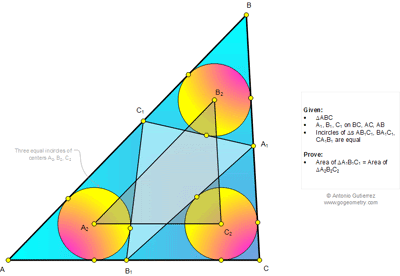## Wednesday, July 30, 2014

### Geometry Problem 1035: Triangle, Three equal Incircles, Tangent lines, Equivalent triangles, Equal area

Level: Mathematics Education, High School, Honors Geometry, College.

Click the figure below to view the complete problem 1035.1.As we had proved in problem 1034, the perimeters are equal so by applying Heron's theorem
in both of the triangles,it will give the same result.

1.To Dima
Problem 1035
Can you see clearly that the step is correct?
Thanks

2.Let s be the semi-perimeter of the two triangles of equal perimeter and r is the radius of the three circles congruent.
S1 is the area of triangle A1B1C1 and S2 the area of triangle A2B2C2.
In addition, S is the area of the hexagon A1B2C1A2B1C2 and A (A1B2C1) is the area of the triangle A1B2C1.
S = S1 + A (A1B2C1) + A (C1A2B1) + A (B1C2A1) = S1 + r s
S = S2 + A (C2A1B2) + A (B2C1A2) + A (A2B1C2) = S2 + r s
From the comparison it follows: S1 = S2.

1.You have a nice and simple solution.
Peter Tran